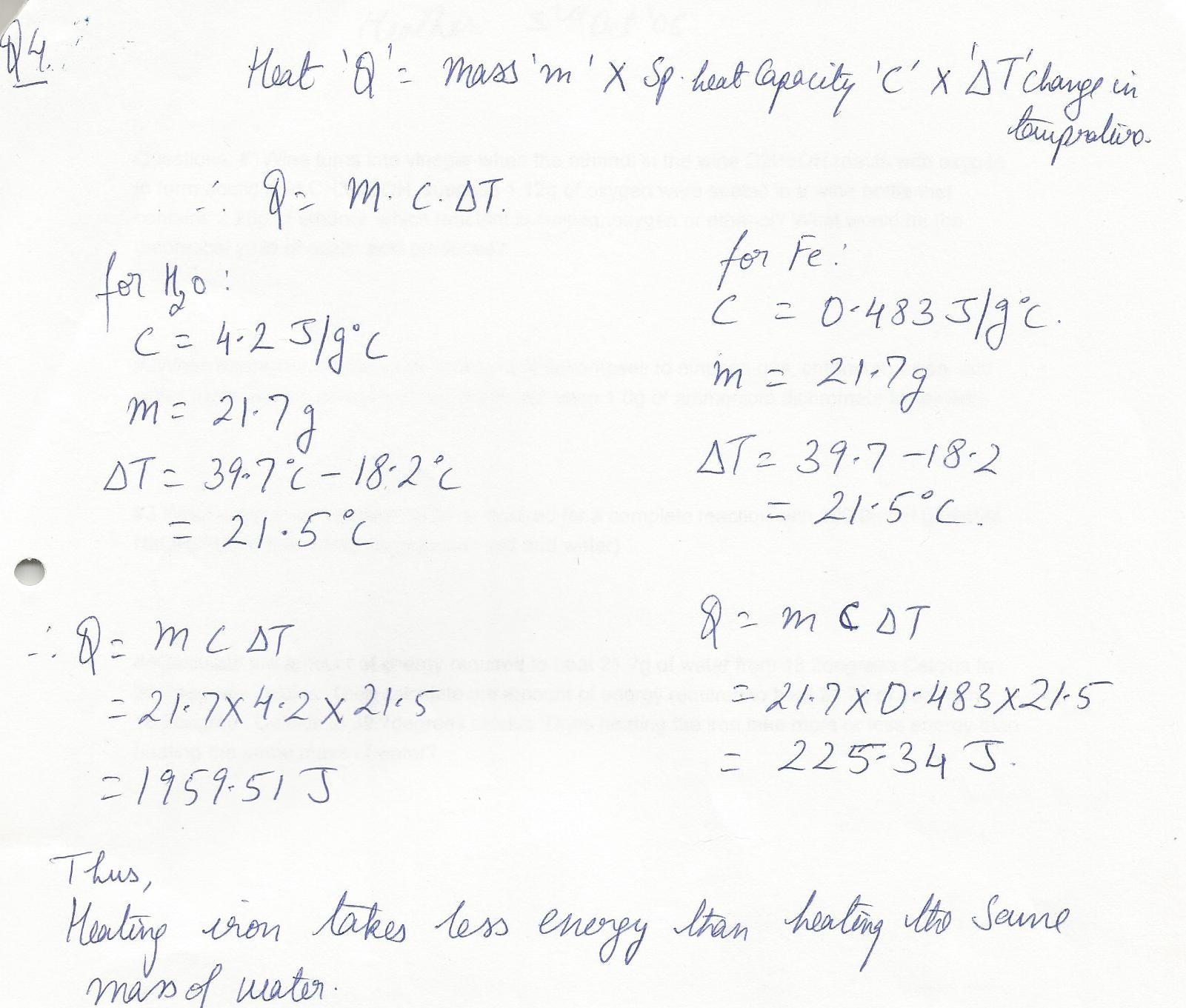8110

Physics Heat & Thermodynamics Level: Misc Level

Calculate the amount of energy required to heat 21.7 g of water from 18.2 degrees Celcius to 39.7 degrees celcius.Then calculate the amount of energy required to heat 21.7 g of iron from 18.2 degrees Celcius to 39.7 degrees celcius. Does heating the iron take more or less energy than heating the same mass of water?